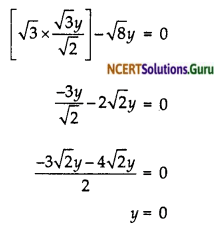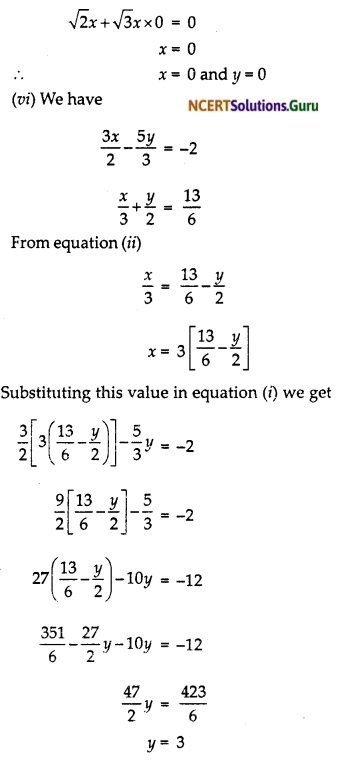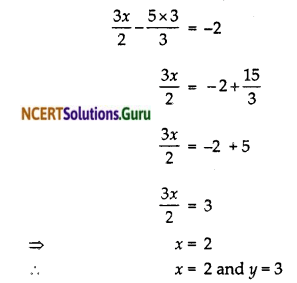# NCERT Solutions for Class 10 Maths Chapter 3 Pair of Linear Equations in Two Variables Ex 3.3

These NCERT Solutions for Class 10 Maths Chapter 3 Pair of Linear Equations in Two Variables Ex 3.3 Questions and Answers are prepared by our highly skilled subject experts.

## NCERT Solutions for Class 10 Maths Chapter 3 Pair of Linear Equations in Two Variables Exercise 3.3Question 1.
Solve the following pair of linear equations by the substitution method,Solution:
(i) The pair of linear equation formed are
x + y = 14 … (i)
x – y = 14 … (ii)
We express x in the term of y from equation (ii) to get
x = y + 4
Now we substitute this value of x in equation (i) we get
(y + 4) + y = 14
2y = 14 – 4
y = 5
Putting this value of equation (ii)
x – 5 = 4
x = 9
∴ x = 9, y = 5

(ii) We have
s – t = 3 … (i)
$$\frac { s }{ 3 }$$ + $$\frac { t }{ 2 }$$ = 6 … (ii)
We express s in the terms of t from equation (i)
s = 3 + t
2t + 6 + 3t = 36
5t = 30
t = 6
Putting this value in equation (i)
s – 6 = 3
s = 9
∴ t = 6 and s = 9

(iii) We have
3x – y = 3 … (i)
9x – 3y = 9 … (ii)
From equation (i)
y = 3x – 3
Substituting this value in equation (ii) we get
9x – 3(3x – 3) = 9
9x – 9x + 9 = 0
9 = 9
The statement is true for all value of y
So y = 3x – 3
Where x can take any value i.e., infinite many solution.

(iv) We have
0.2x + 0.3y = 1.3 …(i)
0.4x + 0.5y = 2.3 …(ii)
From equation (i) we get
x = $$\frac{1.3-0.3 y}{0.2}$$
Substituting this value in equation (ii)
0.4$$\frac{(1.3-0.3 y)}{0.2}$$ + 0.5y = 2.3
2.6 – 0.6y + 0.5y = 2.3
– 0.1y = – 0.3
y = 3
Putting this value in equation (i) we get
∴ 0.2x + 0.3 x 3 = 1.3
0.2x = 1.3-0.9 0.2x = 0.4 x= 2
∴ x = 2 and y = 3

(v) We have
$$\sqrt{2x}$$ + $$\sqrt{3y}$$y = 0 … (i)
$$\sqrt{3x}$$ + $$\sqrt{8y}$$y = 0 … (ii)
From equation (i) we can get
x = $$\frac{-\sqrt{3} y}{\sqrt{2}}$$
Substituting this value in equation (ii)Putting this value in equation (i) we getSubstituting this value in equation (i) we getPutting this value if y if equation (i) we getQuestion 2.
Solve 2x + 3y = 11 and 2x – 4y = – 24 and hence find the value of ‘m’ for which y = mx + 3.
Solution:
We have the two equations
2x + 3y = 11 … (i)
2x – 4y = – 24 … (ii)
From equation (ii)
2x = 4y – 24
x = 2y – 12
Substituting this value in equation (ii)
2(2y – 12) + 3y = 11
4y – 24 + 3y = 11
7y = 35
y = 5
Putting this value in equation (i)
2x + 15 – 11 x = – 2
Putting these value in
y = mx + 3
we get
5 = – 2m + 3
2 = – 2m
m = – 1
Hence the value of m = – 1 and x = – 2, y = 5

Use this free online round to the nearest mile calculator tool to round off the nearest mile.

Question 3.
Form the pair of linear equations for the following problems and find their solution by substitution method.
(i) The difference between two numbers is 26 and one number is three times the other. Find them.

(ii) The larger of two supplementary angles exceeds the smaller by 18 degrees. Find them.

(iii) The coach of a cricket team buys 7 bats and 6 balls for ₹ 3800. Later, she buys 3 bats and 5 balls for ₹ 1750. Find the cost of each bat and each ball.

(iv) The taxi charges in a city consist of a fixed charge together with the charge for the distance covered. For a distance of 10 km, the charge paid is ₹ 105 and for a journey of 15 km, the charge paid is ₹ 155. What are the fixed charges and the charge per km? How much does a person have to pay for travelling a distance of 25 km?

(v) A fraction becomes 9/11, if 2 is added to both the numerator and the denominator. If, 3 is added to both the numerator and denominator it becomes 5/6, Find the fraction.

(vi) Five years hence, the age of Jacob will be three times that of his son. Five years ago, Jacob’s age was seven times that of his son. What are their present ages?
Solution:
(i) Let 1st number be x and 2nd number be y.
Let x > y
1st condition :
x – y = 26
2nd condition :
x = 3y
Putting x = 3y in equation (i)
3y – y = 26 ⇒ 2y = 26 ⇒ y = 13
From (ii)
x = 3 x 13 = 39
∴ One number is 13 and the other number is 39.

(ii) Let one angle be x and its supplementary angle = y
Let x > y
1st Condition :
x + y = 180°
2nd Condition :
x – y = 18° ⇒ X = 18° + y
From equation (ii), putting the value ofx in equation (i),
18° + y + y = 180° ⇒ 18° + 2y = 180°
2y = 162° ⇒ y = 81°
From (ii) x = 18° + 81° = 99° ⇒ x = 99°
∴ One angle is 81° and another angle is 99°.

(iii) Let the cost of each bat be x
and the cost of each ball by y.
According to question
7x + 6y = 3800 … (i)
3x + 5y = 1750 … (ii)
From equation (i)
3$$\frac{(3800-6 y)}{7}$$ + 5y = 1750
3(3800 – 6y) + 35y = 7 x 1750
17y = 12250 – 11400
y = 50
Putting this value in equation (i)
7x + 300 = 3800
7x = 3500
x = 500
Hence the rate of each but in ₹ 500 and the rate of each ball is ₹ 50

(iv) Let the fixed charge be x
and let the charge per km be y.
Then according to question
x + 10y = 105 … (i)
x + 15 y = 155 … (ii)
From equation (i)
x = 105 – 10y
Substituting this value in equation (ii)
105 – 10y + 15y = 155
5y = 50
y = ₹ 10 per km.
Putting this value in equation (i)
x + 100 = 105
x = ₹ 5
A person travelling distance of 25 km the charges will be.
= x + 25y
= 5 + 25 x 10
= ₹ 255

(v) Let the numerator of the fraction be x
and the denominator of the fraction be y.
Therefore according to question(vi) Let the present age of Jacob be x
and the present age of his son be y
According to question
(x + 5) = 3(y + 5)
Again according to question five years ago
(x – 5) = 7(y – 5)
x – 7y = – 30
From equation (i) we get
x= 3y + 10
Substituting this value in equation (ii)
3y + 10 – 7y = – 30
– 4y = – 40
y = 10
Putting this value in equation (i)
x – 30 = 10
x = 40
Hence the present age of Jacob is 40 years and the present age of his son is 10 years.error: Content is protected !!